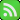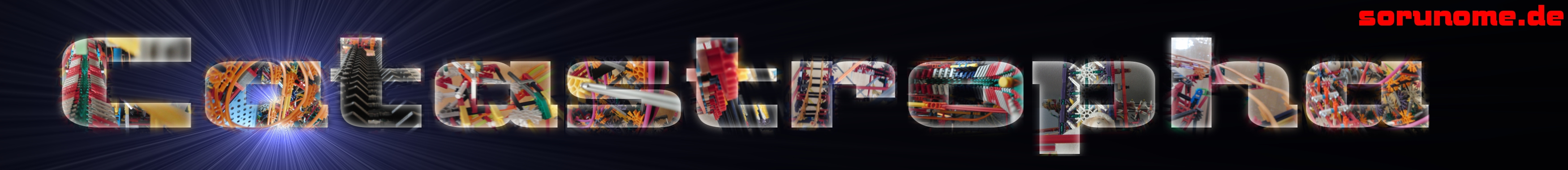(Log In | Register)Construction start 12th October 2010 Construction end 8th January 2012 Number of networks 3 Number of lifts 11 Number of paths 17

This is Catastropha, one of the the largest ball machine I have constructed to date. It has 11 lifts and 17 paths.
The lifts are:
• Wheel lift with holes
• Alternator lift
• Double helix lift
• Ring lift
• Inverted chainsaw lift
• Small arm lift
• Pump list
• Mouse wheel lift
• Inverted motorized single helix lift
• Mill/Wheel lift
• Stair lift
Even thought it might won't seem at the first look, there is equality on Catastropha. The table below will show you.
 Path number Network 1 Network 2 Network 3 1 1/3 0 0 2 0 0 1/6 3 0 0 1/6 4 0 1/3 0 5 1/4 0 0 6 0 0 1/4 7 0 1/4 0 8 0 0 1/4 9 0 1/6 0 11 1/6 0 0 12 1/6 0 0 13 0 1/6 0 14 0 1/12 0 15 1/12 0 0 16 0 0 1/12 17 0 0 1/12 Sum it all up: (1/3)+(1/4)+(1/6)+(1/6)+(1/12)=(4/12)+(3/12)+(2/12)+(2/12)+(1/12)=12/12=1 (1/3)+(1/4)+(1/6)+(1/6)+(1/12)=(4/12)+(3/12)+(2/12)+(2/12)+(1/12)=12/12=1 (1/6)+(1/6)+(1/4)+(1/4)+(1/12)+(1/12)=(2/12)+(2/12)+(3/12)+(3/12)+(1/12)+(1/12)=12/12=1

You might wonder why path 10 isn't in the table, that's because that it comes is so rare. To calculate that: It just happens when the four balls leave path eight, as it have to be four we have to do (1/4)*(1/4)=(1/16). And now only one of the balls go down that track so (1/16)*(1/4)=(1/64).